1332.0.55.002 - Statistical Language!, 2008
ARCHIVED ISSUE Released at 11:30 AM (CANBERRA TIME) 27/06/2008  First Issue
Page tools:Print All

CONTENTS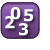Data Includes: Definitions, Example Data are observations or facts that can become information or knowledgeIndex Includes: Definition:, What do indexes tell you?, How can we calculate an Index?, When is an index not appropriate? An Index is a number used to show the variation in some quantity over time.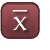Mean Includes: Definition , Here's another way of looking at it!, How do you calculate the mean?, When is the Mean not a useful measure? The mean is a summary number that measures the midpoint of a dataset.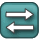Measures of Error and Spread Includes: Definitions, Calculations, Using Standard Deviation, Standard Error and Relative Standard Error Standard deviation measures the scatter in a group of observationsMedian Includes: Definition, What can the Median tell you?, How do you calculate a Median?, When is the Median not a good measure of central tendency? The median is the middle score that seperates the lower half of a dataset from the higher half.Mode Includes: Definition , What does the mode tell you?, How do you calculate a mode?, When is the mode not a good measure of central tendency? The mode is the most commonly observed data item in the data set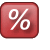Percentage Includes: Definition, What does Percentage tell you?, How do you calculate the Percentage?, Benefits and downfalls of Percentage Percentage is a term used to express a number as a fraction of 100.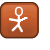Population, Sample and Estimate Includes: Definitions, What do populations and samples tell us?, Which to use in a survey - Population or Sample? A population is any entire group with at least one common characteristic.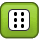Probability Includes: Definition, What can Probability tell you?, How do you calculate Probability?, Benefits and downfalls of Probability Probability refers to the liklihood (or chance) of an event occuring.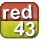Quantitative and Qualitative Includes: Definitions, Example Qualitative data describes an objects attributes that cannot be measured with numbers. Quantitative data is data characterised by numbers.Range Includes: Definition, What does the Range tell you?, How is the Range calculated?, Benefits and downfalls of using the Range Range represents the distance between the highest and lowest values of a data set.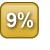Rate Includes: Definition:, What does Rate tell you?, How is the Rate calculated? Rate is an expression of ratio; it represents the relationship between two numbers.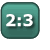Ratio Includes: Definition, What does Ratio tell you?, How do you calculate the Ratio? Ratio is a way of concisely showing the relationship of one quality relative to another.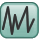Time Series Includes: Definition- Time series, Why are Time-series Created?, Definition- Seasonally Adjusted Time-series, Why are Time-series Seasonally Adjusted?, Definition- Trend Time-series, Why are Trend Time-series Created?, Take care when using Time series A time series is a collection of observations obtained through repeated measurements through time.